3.MD.4.-Test1B Generate measurement data
 Name:    3.MD.4.-Test1B Generate measurement data

Multiple Choice
Identify the choice that best completes the statement or answers the question.

1.

How long is the line to the nearest inch?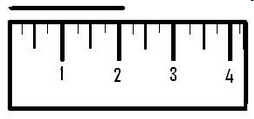a. 1 1/2 in c. 2 1/2 in b. 3 in d. 2 in

2.

What item would be about 1/2 inch long?
 a. marker c. shoe b. fingernail d. calculator

3.

What item would be about 60 inches tall?
 a. flower c. adult b. ant d. house

4.

What item would be about 2 inches long?
 a. eraser c. cup b. pen d. book

5.

How long is the line to the nearest 1/4 inch?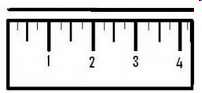a. 4 in c. 5 in b. 4 1/2 d. 4 1/4 in

6.

How long is the line to the nearest 1/4 inch?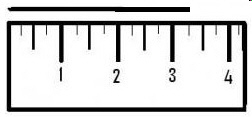a. 3 1/4 in c. 2 3/4 in b. 3 1/2 in d. 3 in

7.

How long is the line to the nearest 1/2 inch?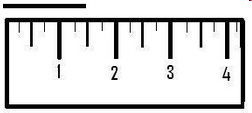a. 1/2 in c. 1 1/2 in b. 2 in d. 1 in

8.

How long is the line to the nearest 1/2 inch?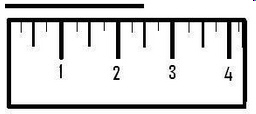a. 1/2 in c. 1 1/2 in b. 2 in d. 2 1/2 in

9.

How long is the line to the nearest inch?a. 2 in c. 2 1/2 in b. 3 in d. 3 1/2 in

10.

What item would be about 8 inches long?
 a. penny c. foot b. pea d. gum